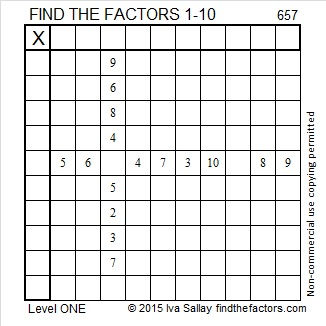# 657 and Level 1

657 is made from three consecutive numbers and the number in the middle, 6, is divisible by 3 so 657 is divisible by both 3 and 9.

657 is the hypotenuse of the Pythagorean triple 432-495-657. What is the greatest common factor of those three numbers?Print the puzzles or type the solution on this excel file: 10 Factors 2015-10-26

—————————————————————————————————

• 657 is a composite number.
• Prime factorization: 657 = 3 x 3 x 73, which can be written 657 = (3^2) x 73
• The exponents in the prime factorization are 2 and 1. Adding one to each and multiplying we get (2 + 1)(1 + 1) = 3 x 2  = 6. Therefore 657 has exactly 6 factors.
• Factors of 657: 1, 3, 9, 73, 219, 657
• Factor pairs: 657 = 1 x 657, 3 x 219, or 9 x 73
• Taking the factor pair with the largest square number factor, we get √657 = (√9)(√73) = 3√73 ≈ 25.632011.—————————————————————————————————This site uses Akismet to reduce spam. Learn how your comment data is processed.